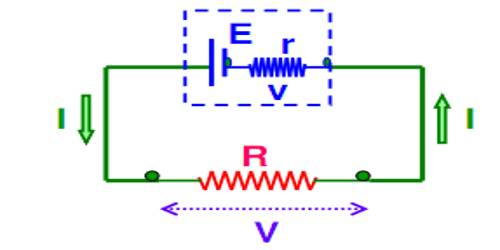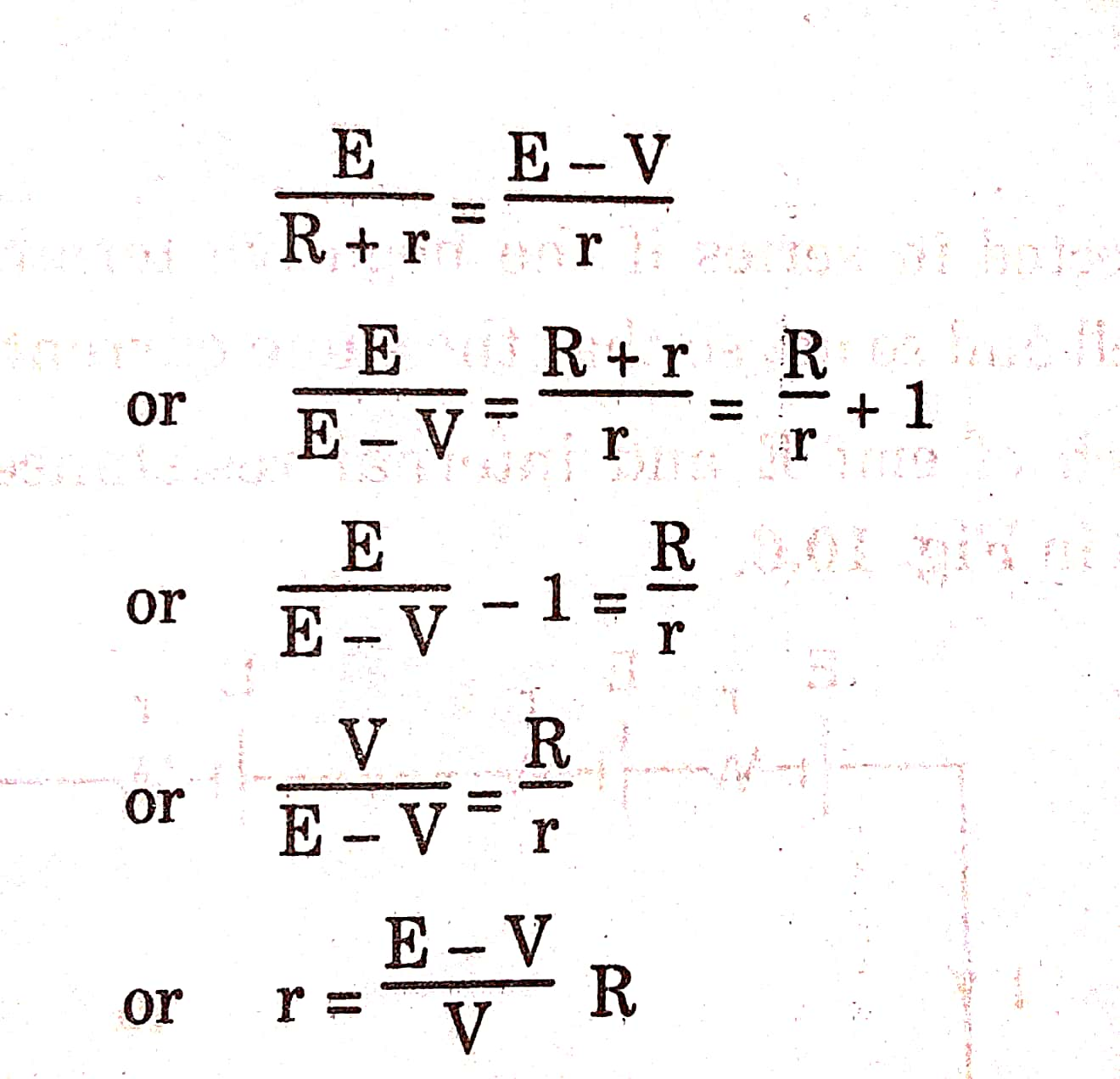Circuit FormulaConsider a cell of emf E and internal resistance r connected to a resistance R in a circuit as shown in Fig. 10.4. A steady current flows through the circuits. Since p.d. across R is IR and potential difference across internal resistance r is 1 r, then

E = IR+Ir

or,    E=I(R+r)

I= E/(R+r)…………..1

This is the current in the circuit. As the external resistance R is connected across the terminals of the cell, so, terminal the potential difference, V potential difference across R

or V=IR……………..2

E IR+Ir= V+Ir

or V=E-Ir……………Figure 10.4 A current flowing through a closed circuit

This is a useful formula for terminal potential differences. In an open circuit, I = 0 so,

V=E-0r=E

So, the emf of the cell in an open circuit is equal to its terminal p.d.

The current in the circuit can be obtained as

From Eqs. (i) and (iv), we haveThis is the relation between emf, terminal p.d. and internal resistance of a cell.

Knowing E, V, and R the value of r can be calculated.